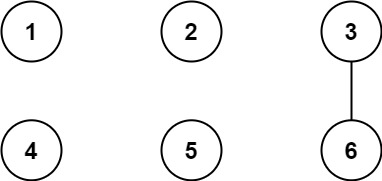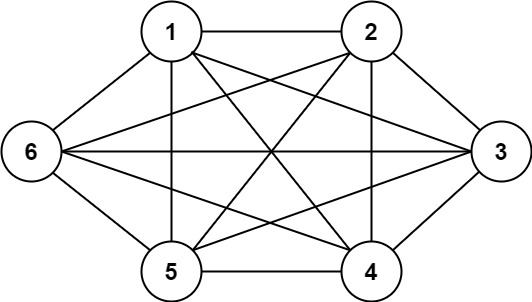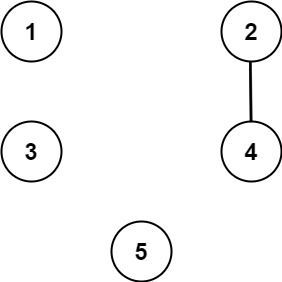1627. Graph Connectivity With Threshold
Hard
490
28

We have `n` cities labeled from `1` to `n`. Two different cities with labels `x` and `y` are directly connected by a bidirectional road if and only if `x` and `y` share a common divisor strictly greater than some `threshold`. More formally, cities with labels `x` and `y` have a road between them if there exists an integer `z` such that all of the following are true:

• `x % z == 0`,
• `y % z == 0`, and
• `z > threshold`.

Given the two integers, `n` and `threshold`, and an array of `queries`, you must determine for each `queries[i] = [ai, bi]` if cities `ai` and `bi` are connected directly or indirectly. (i.e. there is some path between them).

Return an array `answer`, where `answer.length == queries.length` and `answer[i]` is `true` if for the `ith` query, there is a path between `ai` and `bi`, or `answer[i]` is `false` if there is no path.

Example 1:```Input: n = 6, threshold = 2, queries = [[1,4],[2,5],[3,6]]
Output: [false,false,true]
Explanation: The divisors for each number:
1:   1
2:   1, 2
3:   1, 3
4:   1, 2, 4
5:   1, 5
6:   1, 2, 3, 6
Using the underlined divisors above the threshold, only cities 3 and 6 share a common divisor, so they are the
only ones directly connected. The result of each query:
[1,4]   1 is not connected to 4
[2,5]   2 is not connected to 5
[3,6]   3 is connected to 6 through path 3--6
```

Example 2:```Input: n = 6, threshold = 0, queries = [[4,5],[3,4],[3,2],[2,6],[1,3]]
Output: [true,true,true,true,true]
Explanation: The divisors for each number are the same as the previous example. However, since the threshold is 0,
all divisors can be used. Since all numbers share 1 as a divisor, all cities are connected.
```

Example 3:```Input: n = 5, threshold = 1, queries = [[4,5],[4,5],[3,2],[2,3],[3,4]]
Output: [false,false,false,false,false]
Explanation: Only cities 2 and 4 share a common divisor 2 which is strictly greater than the threshold 1, so they are the only ones directly connected.
Please notice that there can be multiple queries for the same pair of nodes [x, y], and that the query [x, y] is equivalent to the query [y, x].
```

Constraints:

• `2 <= n <= 104`
• `0 <= threshold <= n`
• `1 <= queries.length <= 105`
• `queries[i].length == 2`
• `1 <= ai, bi <= cities`
• `ai != bi`
Accepted
14.3K
Submissions
30.9K
Acceptance Rate
46.4%

Seen this question in a real interview before?
1/4
Yes
No

Discussion (0)

Related Topics